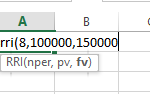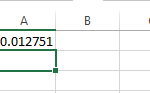### RRI

RRI is a new function that was introduced in Excel 2013. It returns the equivalent interest rate for the growth of an investment.  The inputs required are the number of periods, the present value and the future value.
As an example, let’s say you invested \$100,000 for 8 years compounded annually and the investment grows to a value of \$150,000.  What is the equivalent rate of return?  In these examples, we will type the formula directly into the cell and not use the function wizard.
Start in the cell with =RRI( and then type the inputs as shown below.Remember, compounding periods are important.  What if the investment was for 8 years compounded quarterly?  You would need to adjust NPER for the quarterly compounding so it would be 8 years x 4 compounding periods per year = 32.  You will also need to adjust the result if the desired answer is an annual rate.  You can type a new formula by starting with =RRI( and then typing the new inputs or selecting the cell and pressing F2 to edit the existing formula.